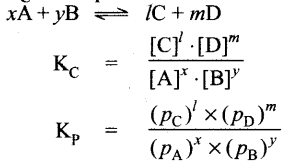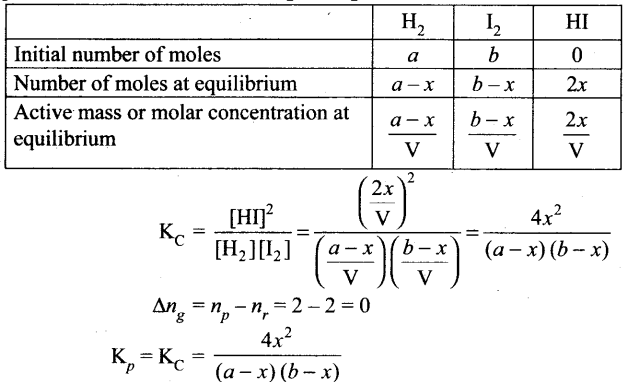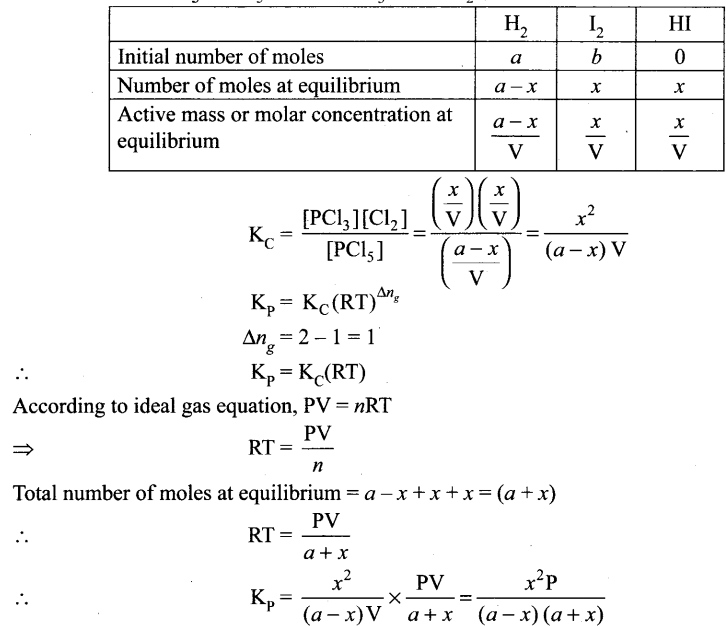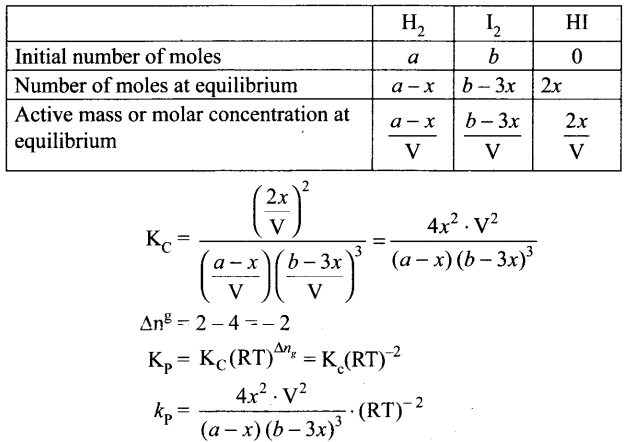## Tamilnadu Samacheer Kalvi 11th Chemistry Notes Chapter 8 Physical and Chemical Equilibrium Notes

Physical equilibrium: A system in which the amount of matter constituting different phases does not change with time is said to be in physical equilibrium.

Solid-liquid equilibrium: H2O(s) ⇌ H2O(l)
At equilibrium, Rate of melting of ice = Rate of freezing of water

Liquid-vapour equilibrium: H2O(l) ⇌ H2O(g)
At equilibrium, Rate of evaporation = Rate of condensation

Solid-Vapour equilibrium: I2(s) ⇌ I2(g)

At equilibrium, Rate of sublimation = Rate of deposition

Equilibrium involving dissolution of solid in liquid: Sugar (solid) ⇌ Sugar (solution) At equilibrium, Rate of dissolution of solute = Rate of crystallisation of solute

Gas in liquid equilibrium: C2 (g) ⇌ CO2 (solution)

At equilibrium, Rate of vaporisation = Rate of condensation

Chemical equilibrium: In a chemical reaction, chemical equilibrium is the state in which both reactants and products are present in concentration which have no further tendency to change with time.

At chemical equilibrium,
Rate of forward reaction (Rf) = Rate of reverse reaction (Rr)

Dynamic equilibrium: At equilibrium, the forward and the backward reactions are proceeding at the same rate and no macroscopic change is observed. So chemical equilibrium is in a state of dynamic equilibrium.

Homogeneous equilibrium: In this equilibrium, all the reactants and products are in the same phase.
H2(g) + I2(g) ⇌ 2HI(g)

Heterogeneous equilibrium: If the reactants and products of a reaction in equilibrium are in different phases, then it is called as heterogeneous equilibrium.
CaCO3(s) ⇌ CaO (s) + CO2(g)

Law of mass action: This law states that “At any instant, the rate of a chemical reaction at a given temperature is directly proportional to the product of the active masses of the reactants at that instant.

Active mass: [n/V]mol dm-3 (or) mol L-1 where “w” is the number of moles of substance and V is the volume of the substance in dm3 or L.

Equilibrium constants Kc and KpRelation between Kp and KC : KP = KC (RT)Δng

Equilibrium constant for heterogeneous equilibrium:
CaCO3(s) ⇌ CaO(s) + CO2(g)
KC – [CO2], Kp – pCo2

Application of equilibrium constant: It is used to predict the direction in which the net reaction will take place, predict the extent of the reaction, calculate the equilibrium concentrations of reactants and products.

Reaction quotient, Q: Under non equilibrium conditions it is defined as the ratio of the product of active masses of reaction products raised to the respective stoichiometric coefficients in the balanced chemical equation to that of the reactants.
$$\frac{[\mathrm{C}]^{l}[\mathrm{D}]^{m}}{[\mathrm{~A}]^{x}[\mathrm{~B}]^{y}}$$
Under non equilibrium conditions.

If Q = KC, the reaction is in equilibrium state. If Q > KC, the reaction will proceed in the reverse direction, i.e., formation of reactants. If Q < KC, the reaction will proceed in the forward direction, i.e., formation of products.

Kp and Kc values for synthesis of HI: H2(g) + I2(g) ⇌ 2HI(g)Dissolution of PCl: PCl(g) ⇌ PCl3(g) + Cl2Synthesis of amonia: N2(g) + 3H3(g) ⇌ 2NH3Le-Chatlier’s principle: “If a system at equilibrium is disturbed, then the system shifts itself in a direction that nullifies that effect of that disturbance.”

Effect of concentration : Increase in concentration of a substance is to shift the equilibrium in a direction that consumes the added substance and vice-versa.

Effect of pressure: When pressure of an equilibrium system having gaseous components is increased then the equilibrium shift in the direction that has fewer moles of gaseous components and vice-versa.

Effect of temperature: If the temperature of an equilibrium system increases then the equilibrium shift in the direction that consumes heat and vice-versa.

Effect of catalyst: It does not affect the state of equilibrium. However, it speed up the attainment of equilibrium by lowering activation energy.

Effect of inert gas: When an inert gas is added to an equilibrium system at constant volume then it has no effect on equilibrium.

Van’t Hoff equation: ∆G° = – RT/wK and ∆G° = ∆H° – T∆S° ⇒ – RTlnK = ∆H° – T∆S° where K = equilibrium constant , ∆G° = free energy change, ∆H° = Enthalpy change, AS° = Entropy change

Differential form of van’t Hoff equation: $$\frac{d(\ln \mathrm{K})}{d \mathrm{~T}}=\frac{\Delta \mathrm{H}^{\circ}}{\mathrm{RT}^{2}}$$

Integrated form of van’t Hoff equation: $$\log \frac{\mathrm{K}_{2}}{\mathrm{~K}_{1}}=\frac{\Delta \mathrm{H}^{\circ}}{2.303 \mathrm{R}}\left[\frac{\mathrm{T}_{2}-\mathrm{T}_{1}}{\mathrm{~T}_{2} \mathrm{~T}_{1}}\right]$$

Samacheer Kalvi 11th Chemistry Notes